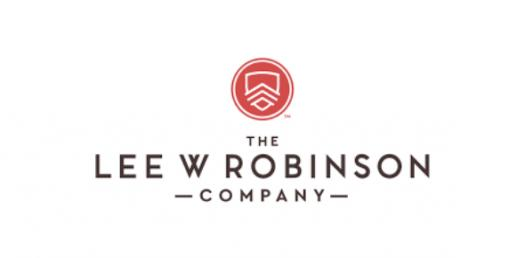# Measurement Quiz Ultimate Trivia Questions!

25 Questions | Total Attempts: 35SettingsThis measurement quiz is an interesting test on measures and weights. The use of images and regularly used products makes it even more appealing. Take it up and gauge how good you are with weights.

Related Topics
• 1.
A bag of potatoes weighs about:
• A.

10 ounces

• B.

10 pounds

• C.

100 pounds

• D.

10 tons

• 2.
A can of coffee weighs about:
• A.

5 ounces

• B.

50 ounces

• C.

5 pounds

• D.

5 tons

• 3.
Jessica made one container of berry punch and served it to her friends at a picnic.  The container served 8 people.  What would be a reasonable amount of liquid in the container?
• A.

0.5 liters

• B.

1 liters

• C.

2 liters

• D.

18 liters

• 4.
Mandy recieved a samll box from the mailman.  What is a reasonable weight for the small box?
• A.

5 grams

• B.

5 pounds

• C.

15 millimeters

• D.

50 inches

• 5.
Kendra wanted to take a bubble bath.  What is a reasonable estimate for the capacity of her bathtub?
• A.

20 cups

• B.

2 quarts

• C.

2 gallons

• D.

20 gallons

• 6.
Which unit of measurement is the most reasonable to measure the length of Dalton's hamster?
• A.

Inches (in)

• B.

Feet (ft)

• C.

Yards (yd)

• D.

Miles (mi)

• 7.
Select the best estimate for the diameter of a quarter:
• A.

0.20 mm

• B.

20 mm

• C.

200 mm

• D.

20 cm

• 8.
Estimate the length of a new, unsharpened pencil
• A.

18 mm

• B.

8 cm

• C.

18 cm

• D.

8 m

• 9.
How many fluid ounces are in 4 quarts?Customary Capacity:1 gallon = 4 quarts1 quart= 2 pints1 pint= 2 cups1 cup = 8 fluid ounces1 gallon = 128 fluid ounces
• A.

16 fl. oz

• B.

32 fl. oz

• C.

64 fl. oz

• D.

128 fl. oz

• 10.
Choose the symbol to make the following statement true.    3 lb.  ________  32 oz.
• A.

>

• B.

• C.

=

• D.

None of the above

• 11.
Kevin is baking a cake for his math class.  The recipe calls for 40 milliliters of water for every 20 milliliters of cooking oil.  How many mililiters of water are needed if 50 milliliters of cooking oil were used?
• A.

25 ml

• B.

100 ml

• C.

200 ml

• D.

1,000 ml

• 12.
Which conversion requires multiplication?
• A.

Decimeter ===> Meter

• B.

Meter ===> Kilometer

• C.

Kilometer ===> Meter

• D.

Millimeter ===> Centimeter

• 13.
Which conversion requires division?
• A.

Milligram ===> Centigram

• B.

Kilogram ===> Gram

• C.

Centigram ===> Milligram

• D.

Gram ===> Milligram

• 14.
Choose the correct conversion of 42 grams to milligrams.
• A.

0.042

• B.

0.42

• C.

420

• D.

42,000

• 15.
Choose the set of measurements listed in the order from least to greatest.
• A.

5 g, 5 cg, 5 mg

• B.

5 g, 5 mg, 5 kg

• C.

5 mg, 5 g, 5 kg

• D.

5 mg, 5 kg, 5 g

• 16.
What is the measurement to the nearest tenth of a centimeter of this line segement?
• A.

3 cm

• B.

3 and 1/10 cm

• C.

3 and 3/10 cm

• D.

3 and 7/10

• 17.
What is the measurement to the nearest sixteenth of an inch in this line segment?
• A.

4 inches

• B.

4 and 1/16 inches

• C.

4 and 3/16 inches

• D.

4 and 7/16 inches

• 18.
What is the exact measurement of the line segment?
• A.

3 and 1/4 inches

• B.

2 and 1/2 inches

• C.

3 and 5/8 inches

• D.

2 and 3/4 inches

• 19.
In degrees Celsius, what is the temperature shown on the thermometer?
• A.

12 degrees Celsius

• B.

17 degrees Celcius

• C.

63 degrees Celcius

• D.

65 degrees Celcius

• 20.
What is the exact temperature in degrees Fahrenheit as shown on the thermometer?
• A.

12 degrees F

• B.

15 degrees F

• C.

48 degrees F

• D.

53 degrees F

• 21.
Which of the following could you measure with a balance scale?
• A.

Height of a room

• B.

Amount of water in a swimming pool

• C.

Weight of sugar

• D.

Distance between two cities

• 22.
What is the length of this string?
• A.

20 cm

• B.

30 cm

• C.

50 cm

• D.

70 cm

• 23.
What is the length of this pencil?
• A.

3 inches

• B.

3.5 inches

• C.

4 inches

• D.

4.5 inches

• 24.
This ruler shows length in centimeters.  What is the length of this ribbon?
• A.

12.5 in

• B.

6 cm

• C.

13 cm

• D.

14 in

• 25.
Type response below